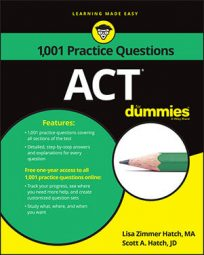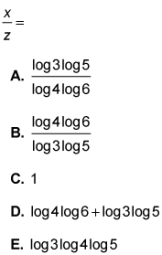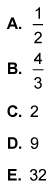##### ACTIf you think a logarithm is a tree that can do the Macarena, you may want to do some studying before you take the ACT Math exam. Then, you can come back and tackle the following practice questions, where you have to use the properties of logarithms to solve two different equations.

## Practice questions

1. If 3x = 4y and 5y = 6z, then2. For which of following values for x is log64 + log6x = 2?1. The correct answer is Choice (B).

Looking at the answer choices tells you you're dealing with a logarithm problem. Because you're solving for

and none of the answers has a y variable, you need to get rid of the y variables in the original equations. You can do this by solving both equations for y and them setting them equal to each other. Then, you can manipulate the equation to solve for

First, take the log of both sides of the first equation and solve for y:

Then, take the log of both sides of the other equation and solve for y:

Set the equations equal to each other and move terms around until you've solved for x/z:

2. The correct answer is Choice (D).

When you add logs with the same base, you solve by multiplying: log6(4x) = 2.

Then you can plug in the options for x. Choice (D) is correct. The product of 4 and 9 is 36, which is the value you get when you multiply 6 by itself two times.

Choice (E) incorrectly adds 4 and x.